# Visualization of large datasets with tabplot

The tableplot is a powerful visualization method to explore and analyse large multivariate datasets. In this vignette, the implementation of tableplots in R is described, and illustrated with the diamonds dataset from the ggplot2 package.

## Introduction

The tableplot is a visualization method that is used to explore and analyse large datasets. Tableplots are used to explore the relationships between the variables, to discover strange data patterns, and to check the occurrence and selectivity of missing values.

A tableplot applied to the diamonds dataset of the ggplot2 package (where some missing values were added) is illustrated in the figure below. Each column represents a variable. The whole data set is sorted according to one column (in this case, carat), and then grouped into row bins.

Tableplots are aimed to visualize multivariate datasets with several variabels (up tot a dozen) and a large number of records, say at least one thousand. Tableplots can also be generated for datasets with less records, but they may be less useful. The maximum number of rows that can be visualized with the tabplot package depends on the R's memory, or, when using the ff package, on the limitations of that package.

## Getting started with the tableplot function

The diamonds dataset is very suitable to demonstrate the tabplot package. To illustrate the visualization of missing values, we add several NA's.

require(ggplot2)
data(diamonds)
is.na(diamonds$price) <- diamonds$cut == "Ideal"
is.na(diamonds$cut) <- (runif(nrow(diamonds)) > 0.8)  A tableplot is simply created by the function tableplot. By default, all variables of the dataset are depicted. With the argument select, we can specify which variables are plotted. The dataset is by default sorted according to the values of the first column. With the argument sortCol, we can specify on which column(s) the data is sorted. tableplot(diamonds)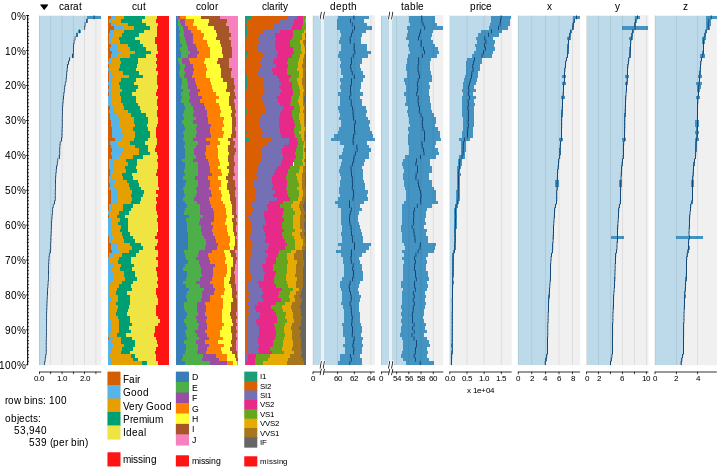The tableplot below consists of five columns, where the data is sorted on price. Notice that the missing values that we have added are placed at the bottom and (by default) shown in a bright red color. tableplot(diamonds, select = c(carat, price, cut, color, clarity), sortCol = price)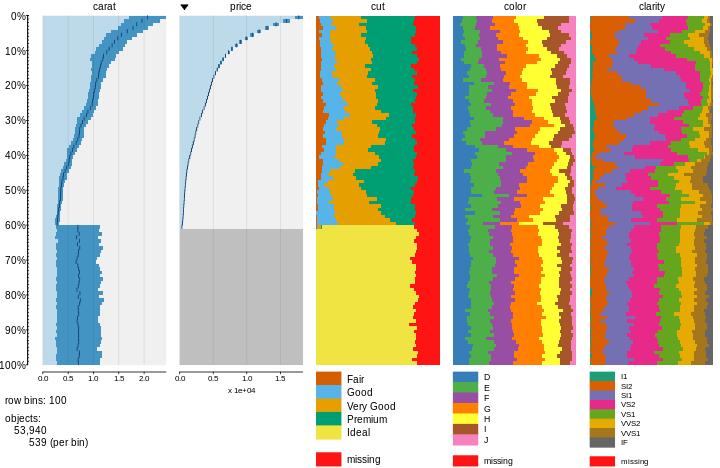Setting an appropriate number of row bins (with the argument nBins) is important, like in a histogram. A good number of row bins is a trade of between good polished but meaningless data, and detailed, but noisy data. In practice, we found that the default number of 100 usually is a good starting point. The percentages near the vertical axis indicate which subset of the data in terms of units (rows) is depicted. The range from 0% to 100% in the figure above means that all units of the data are plotted. ## Zooming and filtering ### Zooming We can focus our attention to the 5% most expensive diamonds by setting the from argument to 0 and the to argument to 5. The resulting tableplot are depicted in the figure below. Observe that the number of row bins is still 100, so that the number of units per row bin is now 27 instead of 540. Therefore, much more detail can be observed in this tableplot. tableplot(diamonds, select = c(carat, price, cut, color, clarity), sortCol = price, from = 0, to = 5)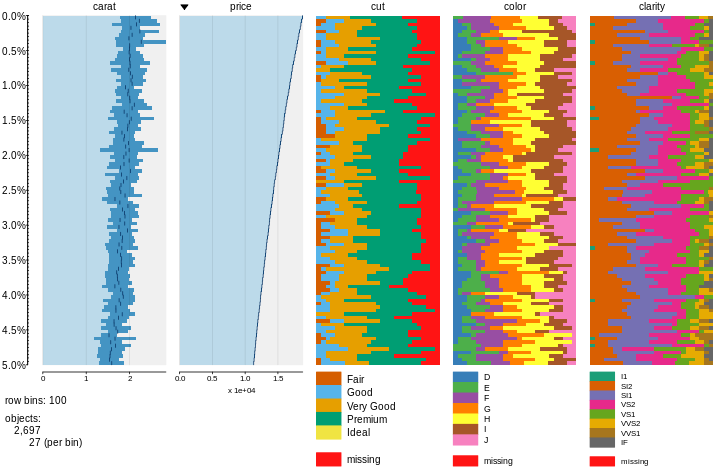The vertical axis contains two sets of tick marks. The small tick marks correspond with the row bins and the large tick marks correspond with the percentages between from and to. ### Filtering The argument subset serves as a data filter. The tableplot in the following figure shows that data of premium cut diamonds that cost less than 5000$.

tableplot(diamonds, subset = price < 5000 & cut == "Premium")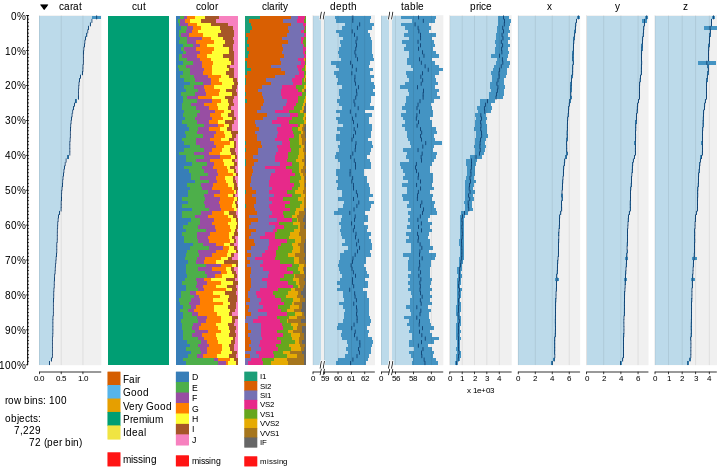It is also possible to create a tableplot for each category of a categorical variable in one call. For instance, by setting subset=color we create a tableplot for each color class.

## Continuous variables

### Scaling

For each bin of a continuous variable, the mean value and the standard deviation are calculated. When the distribution of these mean values is exponential, it is useful to apply a logarithmic transformation. The argument scales can be set to linear mode "lin", logarithmic mode "log", or the default value "auto", which automatically determines which of the former two modes is used.

### Plotting mode and used colors

The argument numMode can be passed on tableplot. It determines how the numeric variables are plot:

1. "MB-ML" (default of versions prior to 1.2). The colors of the bins indicate the fraction of missing values. A diverging color palette is used, which is specified by numPals. By default, if a bin does not contain any missing values, the corresponding bar is depicted in dark. The more missing values, the brighter the color. For positive mean values, the right-hand side of the palette is used, and for negative, the left-hand side. The more missing values, the brighter the color. Bars of which all values are missing are depicted in light red.
2. mb-sdb-ml (default). Numeric variables are plot in colors of the right-hand side of the palette specified by numPals, by default blues. Mean bars are plot in light blue, standard deviation bars in medium blue, and mean vertical lines in dark blue.
tablePalettes()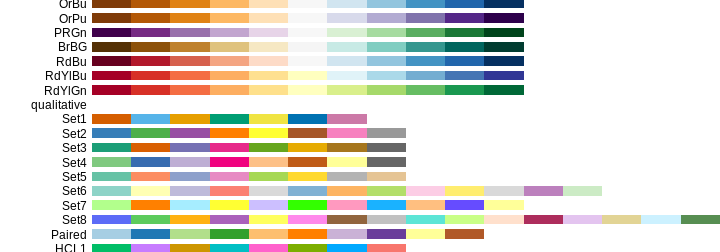### X-axes

The x-axes a plotted as compact as possible. This is illustrated in the x-axis for the variable price.

Observe that the x-axes of the variables depth and table in the top figure are broken. In this way the bars are easier to differentiate. The argument bias_brokenX can be set to determine when a broken x-axis is applied.

For each numerical variable, the limits of the x-axes can be determined manually with the argument limitsX.

## Categorical variables

### Color palettes

The implemented palettes are depicted in the figure above. These palettes, as well as own palettes, can be assigned to the categorical variables with the argument pals.

Suppose we want a to use the default palette for the variable cut, but starting with the sixth color, blue. Further we want the fifth palette for the variable color, and a custom palette, say a rainbow palette, for the variable clarity. The resulting tableplot is depicted in Figure~\ref{fig:tp5.

tableplot(diamonds, pals = list(cut="Set1(6)", color="Set5", clarity=rainbow(8)))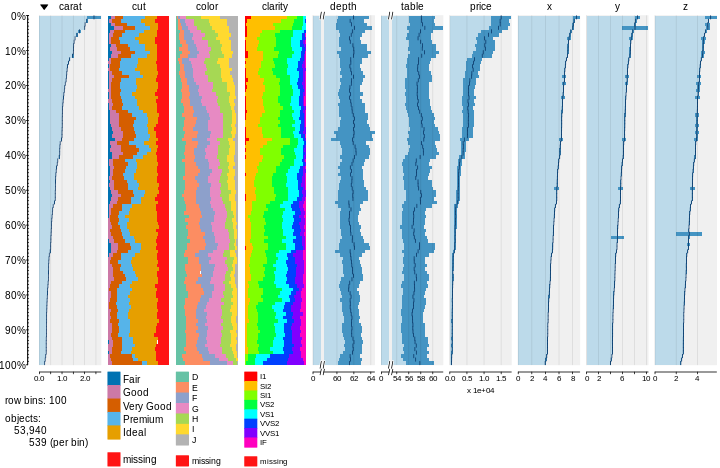Also quantitative palettes can be used (for instance by setting clarity= "Greens". Missing values are by default depicted in red. This can be changed with the argument colorNA.

### High cardinality data

To illustrate how tableplots deal with high cardinality data, we extend the diamonds dataset with a convenient wrapper function num2fac:

diamonds$carat_class <- num2fac(diamonds$carat, n=20)
diamonds$price_class <- num2fac(diamonds$price, n=100)


For variables with over change_palette_type_at (by default 20) categories, color palettes are constructed by using interpolated colors. This creates a rainbow effect. If the number of categories is than change_palette_type_at, the assigned palette is recycled in order to obtain the number of categories.

tableplot(diamonds, select=c(carat, price, carat_class, price_class))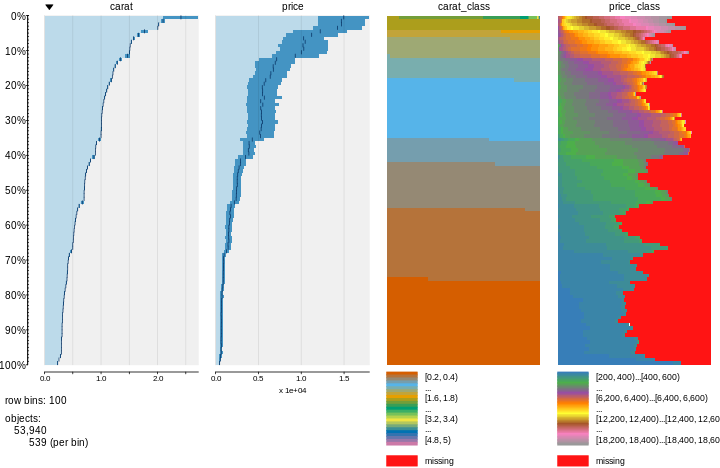If the number of categories exceeds max_level (by default 50), the categories are rebinned into max_level category groups. This is illustrated by the variable price_class.

## Preprocessing of big data

For large datasets it is recommended to preprocess the data with the function tablePrepare. This function determines to sorting order of each data column, the most time consuming task to create tableplots. The advantage of using tablePrepare as an intermediate step, is that the processing time to create tabelplots is reduced big time. This is especially useful when experimenting with arguments such as the number the row bins (nBins) and the sorting column (sortCol), or when using tableplots interactively.

The following example illustrated the tablePrepare function for big data:

# create large dataset
large_diamonds <- diamonds[rep(seq.int(nrow(diamonds)), 10),]

system.time({
p <- tablePrepare(large_diamonds)
})

##    user  system elapsed
##   0.601   0.160   0.761

system.time({
tableplot(p, plot=FALSE)
})

##    user  system elapsed
##   0.170   0.016   0.185

system.time({
tableplot(p, sortCol=price, nBins=200, plot=FALSE)
})

##    user  system elapsed
##   0.175   0.020   0.195


Although the first step takes a couple of seconds on a moderate desktop computer, the processing time to create a tableplot from the intermediate result, object p, is very short in comparison to the direct approach:

system.time({
tableplot(large_diamonds, plot=FALSE)
})

##    user  system elapsed
##   0.767   0.144   0.910

system.time({
tableplot(large_diamonds, sortCol=price, nBins=200, plot=FALSE)
})

##    user  system elapsed
##   0.795   0.151   0.946


## Sampling

To increase the speed even more, it is possible to sample with the argument sampleBinSize:

system.time({
tableplot(p, sample=TRUE)
})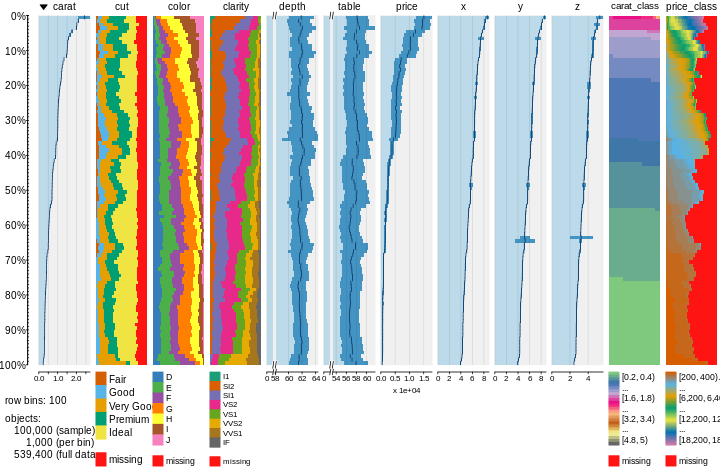##    user  system elapsed
##   0.302   0.000   0.301


## Compare tableplots (experimental)

It is possible to compare two datasets, for instance two samples, two versions of a dataset, or datasets from two different time periods.

# calculate normalized carats to be used as sample probabilities
carat.norm <- with(diamonds, carat / max(diamonds\$carat))

# draw samples
exp.diamonds <- diamonds[sample(1:nrow(diamonds), size=10000, prob=carat.norm, replace=TRUE),]
chp.diamonds <- diamonds[sample(1:nrow(diamonds), size=10000, prob=1-carat.norm, replace=TRUE),]

tp1 <- tableplot(exp.diamonds, plot=FALSE)
tp2 <- tableplot(chp.diamonds, plot=FALSE)

plot(tp2 - tp1)

## Independence of the compared tableplots assumed for the calculation of the standard deviations.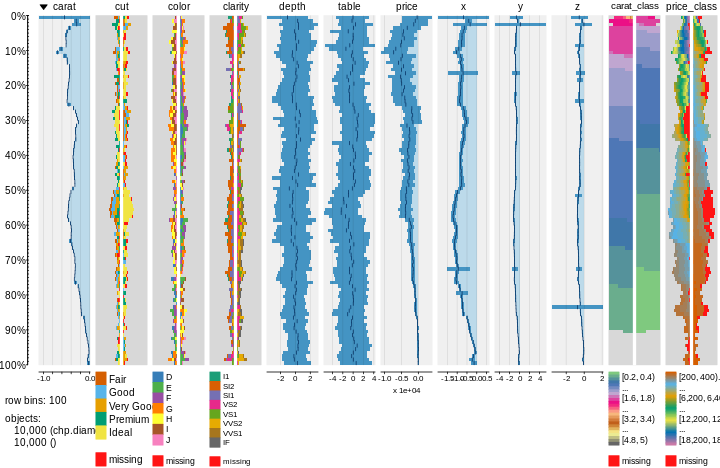This comparison tableplot shows per bin the difference in mean value for each numeric variable, and for each categorical variable a two-sided stacked bar chart to indicate the differences in fractions per category: left-side bars indicate that more items are contained in tp1, right-side bars indicate that more items are contained in tp2, and if bars are not plotted, the fraction of items is unchanged. Relative mean values can be plot with relative=TRUE.

Note: The objects tp1 and tp2 are tabplot-objects (see below). If they are substracted from each other, a tabplot_compare-object is returned.

The comparison treemap is still in development stage, so it may change future updates.

## Miscellaneous

### The tabplot object

The function tableplot returns a tabplot-object, that can be used to make minor changes to the tableplot, for instance the order of columns or the color palettes. Of course, these changes can also be made by generating a new tableplot. However, if it takes considerable time to generate a tableplot, then it is practical to make minor changes immediately.

The output of the tableplot function can be assigned to a variable. The graphical output can be omitted by setting the argument plot to FALSE.

tab <- tableplot(diamonds, plot = FALSE)


The tabplot-object is a list that contains all information to depict a tableplot. The generic functions summary and plot can be applied to the tabplot object.

summary(tab)

##               general               variable1
##  dataset          :diamonds   name       :carat
##  variables        :12         type       :numeric
##  sortCol          :1          scale_init :auto
##  decreasing       :TRUE       scale_final:lin
##  from             :0%
##  to               :100%
##  objects.sample   :53940
##  objects.full.data:53940
##  bins             :100
##       variable2                variable3
##  name      :cut           name      :color
##  type      :categorical   type      :categorical
##  categories:6             categories:8
##
##
##
##
##
##
##       variable4                 variable5             variable6
##  name      :clarity       name       :depth     name       :table
##  type      :categorical   type       :numeric   type       :numeric
##  categories:9             scale_init :auto      scale_init :auto
##                           scale_final:lin       scale_final:lin
##
##
##
##
##
##        variable7             variable8             variable9
##  name       :price     name       :x         name       :y
##  type       :numeric   type       :numeric   type       :numeric
##  scale_init :auto      scale_init :auto      scale_init :auto
##  scale_final:lin       scale_final:lin       scale_final:lin
##
##
##
##
##
##        variable10           variable11               variable12
##  name       :z         name      :carat_class   name      :price_class
##  type       :numeric   type      :categorical   type      :categorical
##  scale_init :auto      categories:26            categories:51
##  scale_final:lin
##
##
##
##
##

plot(tab)


### Multiple tableplots

When a dataset contains more variables than can be plotted, multiple tableplots can be generated with the argument nCols. This argument determines the maximum number of columns per tableplot. When the number of selected columns is larger than nCols, multiple tableplots are generated. In each of them, the sorted columns are plotted on the lefthand side.

When multiple tableplots are created, the (silent) output is a list of tabplot objects. This is also the case when the dataset is filtered by a categorical variable, e.~g. subset = color (Filtering.

### Layout options

There are several arguments that determine the layout of the plot: fontsize, legend.lines, max_print_levels, text_NA, title, showTitle, fontsize. title, showNumAxes, and vp. The following code illustrates this.

tableplot(diamonds, select = 1:7, fontsize = 14, legend.lines = 8, title = "Shine on you crazy Diamond", fontsize.title = 18)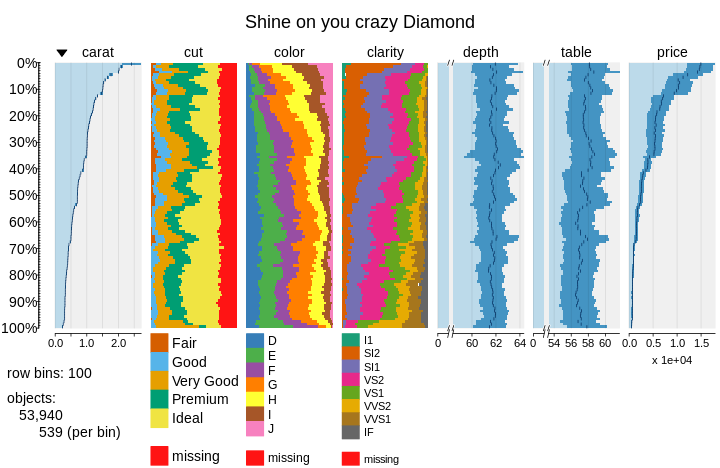The layout arguments named above are passed on from tableplot to plot.tabplot. These arguments will be especially important when saving a tableplot (see Multiple tableplots).

### Minor changes

The function tableChange is used to make minor changes to a tabplot-object. Suppose we want the columns in the order of the top figure, and we want to change all color palettes to default starting with the second color.

tab2 <- tableChange(tab, select_string = c("carat", "price", "cut", "color", "clarity"), pals = list(cut="Set1(2)"))
plot(tab2)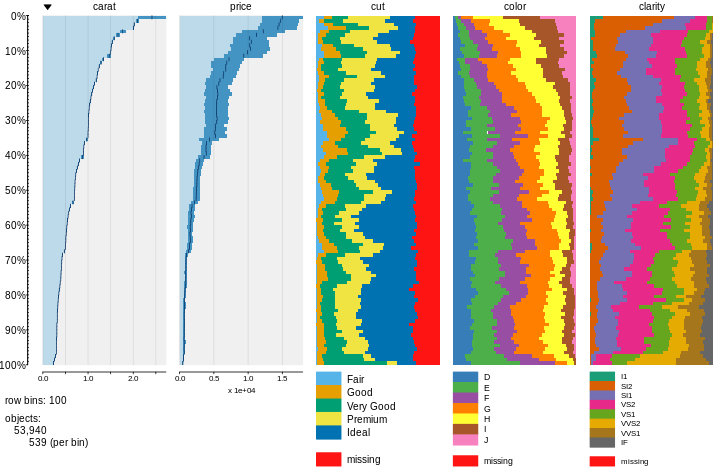### Save tableplots

With the function tableSave, tableplots can be saved to a desired grahical output format: pdf, eps, svg, wmf, png, jpg, bmp, or tiff.

tableSave(tab, filename = "diamonds.png", width = 5, height = 3, fontsize = 6, legend.lines = 6)


All layout options named in Layout options can be used here, such as fontsize and legend.lines. When tab is a list of tabplot-objects (see Multiple tableplots), the argument onePage determines whether the tableplots are stacked on one page or printed on seperate pages.